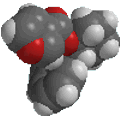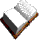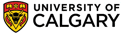Chapter 9 : AlkynesAlkyne Questions

Qu 1: Using the following average bond energies, estimate the strength of the π bonds in an alkyne and an alkene

CC = 820 kJ/mol (196 kcal/mol), C=C = 611 kJ/mol (146 kcal/mol), C-C = 368 kJ/mol (88 kcal/mol)

Qu 2: Consider the following points about the reaction of 2-butyne with HBr

(i) reaction with 1 equivalent gives 2-bromo-2-butene
(ii) reaction with excess gives 2,2-dibromobutane

(a) What conclusions can you reach about the reactivity of 2-bromo-2-butene compared to 2-butyne ?

(b) Consider the two possible carbocations that could be formed in the conversion of 2-bromo-2-butene to 2,2-dibromobutane. Suggest a reason for the observed regioselectivity.

Qu 3: What would be the product from the reaction of propene with following reagent sequence:

(i) Br2 (ii) excess NaNH2 , heat followed by H2O (iii) NaNH2 then MeI

Qu 4: Show the major products, with stereochemistry where applicable, for the reactions of  :

(a) 1-pentyne and (b) 2-pentyne with each of the following :

 (i) H2 / Lindlar Pd (v) excess HCl (ii) excess H2 / Pd (vi) aq. H2SO4, HgSO4 (iii) Na / NH3 (vii) Br2 (iv) 1 equiv. HCl (viii) O3 then H2O© Dr. Ian Hunt, Department of Chemistry